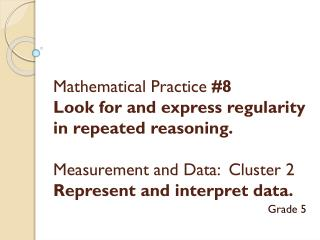DownloadDownload PresentationTélécharger la présentation- - - - - - - - - - - - - - - - - - - - - - - - - - - E N D - - - - - - - - - - - - - - - - - - - - - - - - - - -
##### Presentation Transcript

1. Mathematical Practice #8 Look for and express regularity in repeated reasoning.Measurement and Data: Cluster 2Represent and interpret data. Grade 5

2. Highlighted Problem Ten beakers, measured in liters, are filled with a liquid. The line plot above shows the amount of liquid in liters in 10 beakers. If the liquid is redistributed equally, how much liquid would each beaker have? (This amount is the mean.)

3. Explore Possible Solution Paths There are a total of 3⅛ liters in all 10 beakers. You have to distribute the liters equally to find the number in EACH beaker. NOW WHAT????

4. Draw a Picture - Distribute There are a total of 3⅛ liters in all 10 beakers. You have to distribute the liters equally to find the number in EACH beaker.

5. Draw a Picture - Distribute There are a total of 3⅛ liters in all 10 beakers. You have to distribute the liters equally to find the number in EACH beaker. There are 20 eighths distributed. There are ⅝ liters remaining. ⅛ ⅛ ⅛ ⅛ ⅛ ⅛ ⅛ ⅛ ⅛ ⅛ ⅛ ⅛ ⅛ ⅛ ⅛ ⅛ ⅛ ⅛ ⅛ ⅛

6. Draw a Picture - Distribute There are 10 sixteenths distributed. There are now 2/8 and1/16in each beaker. What is the total amount of liters in each beaker? ⅛ ⅛ ⅛ ⅛ ⅛ ⅛ ⅛ ⅛ ⅛ ⅛ ⅛ ⅛ ⅛ ⅛ ⅛ ⅛ ⅛ ⅛ ⅛ ⅛